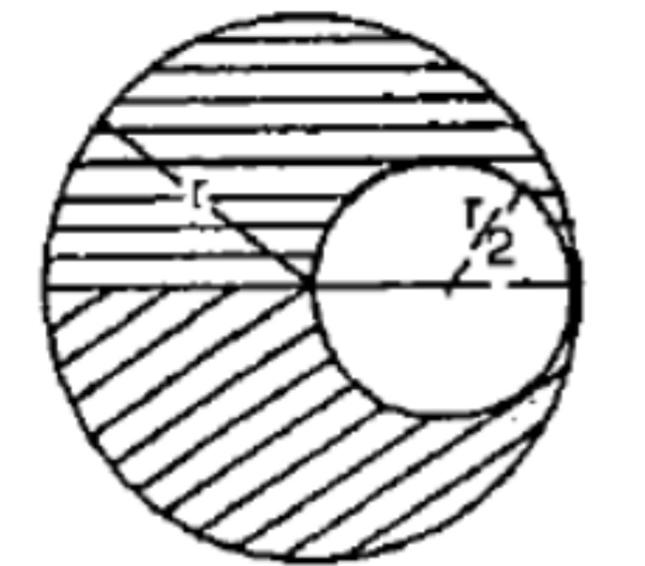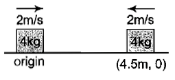#2 | Questions on Center of Mass
(Physics) > Systems of Particles and Rotational Motion
Unable to watch the video, please try another server

#### My Notes

Create your notes while watching video by clicking on icon in video player.
Related Practice Questions :

A circular disc of radius r has a uniform thickness.  A circular hole of diameter equal to the radius of the disc has been cut out as shown.  The centre of gravity of the remaining disc lies on the diameter of the disc at a distance x to the left of the centre of the original disc.  The value of x is(1) r                              (2) $\frac{r}{2}$

(3) $\frac{r}{4}$                           (4) $\frac{r}{6}$

High Yielding Test Series + Question Bank - NEET 2020

Difficulty Level:

In free space, a rifle of mass M shoots a bullet of mass m at a stationary block of mass M at a distance D away from it. When the bullet has moved through a distance d towards the block, the centre of mass of the bullet - block system is at a distance of

(1) $\frac{\left(D-d\right)}{M+m}$ from the bullet

(2) $\frac{md+MD}{M+m}$ from the block

(3) $\frac{2md+MD}{M+m}$ from the block

(4) $\frac{\left(D-d\right)M}{M+m}$ from the bullet

High Yielding Test Series + Question Bank - NEET 2020

Difficulty Level:

Blocks A and B are resting on a smooth horizontal surface given equal speeds of 2 m/s in the opposite sense as shown in the figure.At t = 0, the position of blocks are shown, then the coordinates of centre of mass at t = 3s will be

1.  (1, 0)

2.  (3, 0)

3.  (5, 0)

4.  (2.25, 0)

High Yielding Test Series + Question Bank - NEET 2020

Difficulty Level:

Consider a system of two particles having masses . If the particle of mass ${\mathrm{m}}_{1}$ is pushed towards the center of mass of particles through a distance, by what distance would be the particle of the mass ${\mathrm{m}}_{2}$ move so as to keep the center of mass of particles at the original position?

1.

2.  $\frac{{\mathrm{m}}_{1}}{{\mathrm{m}}_{2}}\mathrm{d}$

3.  d

4.  $\frac{{\mathrm{m}}_{2}}{{\mathrm{m}}_{1}}\mathrm{d}$

High Yielding Test Series + Question Bank - NEET 2020

Difficulty Level:

Consider a system of two identical particles. One of the particles is at rest and the other has an acceleration a. The centre of mass has an acceleration

1. zero

2. 1/2 a

3. a

4. 2 a